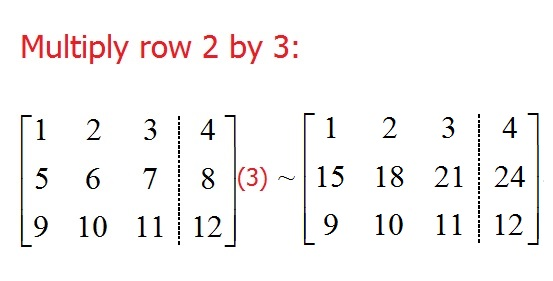# Row operations

Row operations are calculations we can do using the rows of a matrix in order to solve a system of equations, or later, simply row reduce the matrix for other purposes. There are three row operations that we can perform, each of which will yield a row equivalent matrix. This means that if we are working with an augmented matrix, the solution set to the underlying system of equations will stay the same.

## The row operations

In the following examples, the symbol ~ means “row equivalent”.

### Swap two rows

When working with a system of equations, the order you write the questions doesn’t affect the solution. Since an augmented matrix represents a system of equations, with each row being an equation, we can swap two rows.Notice the notation with the double arrow. When you are performing row operations, use notation like this to keep track of what you did. It is very easy to have an arithmetic mistake, and if this happens, this notation let’s you go back and find it easily.

The formal notation for this row operation (as used in some books) would be:$R_1 \leftrightarrow R_3$.

### Multiply a row by a nonzero constant

There will be times when it will be useful to multiply a row by something like 2 or 1/3. Doing this will not change the solution to the underlying system of equations since multiplying any equation by a nonzero constant results in an equivalent equation (as long as you multiply BOTH sides of the equation).In this example, each entry in row 2 was multiplied by the constant. A fair question here would be “Why would you do that row operation?”. We will get into that when we talk about Gauss Jordan elimination and row reduction, but for now, I chose multiplying row 2 by 3 just for the sake of showing you how it would work.

The formal notation for this particular row operation:$3R_2 \rightarrow R_2$. (think: multiply row 2 by 3 and put it back where the original row 2 was)

### Multiply a row by a nonzero constant and add it to another row

Think back to when you first learned how to solve systems of equations. You likely learned how to eliminate a variable by multiplying one equation by a number and then adding the two equations. We can translate that same idea into a row operation.Notice that all the work happened in row 2. Because of this, our shorthand notation has$-5R_1$ next to row 2. To do the actual row operation, we took each value in row 1, multiplied it by –5 and then added it to the corresponding entry in row 2.

The formal notation for this would be:$-5 R_1 + R_2 \rightarrow R2$. (the arrow points to where all the work will go)

## The big picture

The last example shows the true power of row operations. By picking to add$-5R_1$ to row 2, we eliminated$x_1$ in the 2nd equation. If we continued this process and did similar row operations for other variables, then we should be able to eliminate the variables in a way as to see the solution to the underlying system. In fact, this will be exactly what we will study when talking about Gauss Jordan elimination and row reduction.